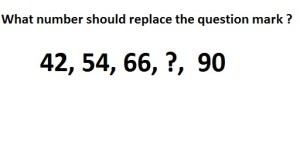Home > Math Shortcuts > Brain Trigger #16 – Sample Number puzzle question

# Brain Trigger #16 – Sample Number puzzle question

Now here we will discuss few question answers on Number puzzle which are very common in competitive exams. These types of Number puzzle questions will help you to prepare for your exam. Try to solve the question mention below using your skills on math. Shortcut tricks can also be used to solve these Number puzzle questions.

Every types of Number puzzle problems and its shortcut tricks, how to do methods, you will find here in this website. Now just apply shortcut tricks of Number puzzle to solve these Number puzzle problems. But you may not need any shortcut tricks to solve some of these questions.

Now try to solve a question on Number puzzle. First of all you need to read the question very carefully and try to solve it by yourself. See below to get the answer of this question. If you do this problem then check the solution of this question with your answer. You can also check below if you don’t know how to do the problem correctly.

A page contain a problem on Number puzzle and also its solution with explanation. Check other questions by clicking on the Next/Previous link below.

Let’s starts the Question Answer session. Today’s question is:Scroll down below to know the solution of this problem. You can take as much time as you want to solve the question. But try to solve this question as quickly as you can.

(Scroll down to get the answer)

(Scroll down to get the answer)

(Scroll down to get the answer)

(Scroll down to get the answer)

Here starts the solution of the above problem. If you try the problem and got an answer then check your solution with us. If you correctly answer the question then Well Done, but don’t worry if you are unable to answer the previous question correctly. Now here is the solution of the problem with explanation.

you can solv this way …………..

33+3×3 = 42
36+3×6 = 54
39+3×9 = 66
42+3×12= 78
45+3×15= 90

 << PREVIOUS QUESTION NEXT QUESTION >>

Here we provide some other questions on different topics also. Click on Next/Previous link, it will take you to different topics.